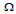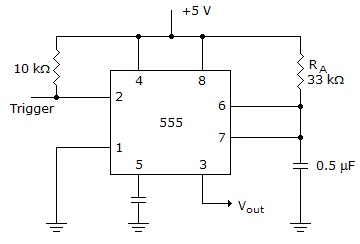# Digital Electronics - Multivibrators and 555 Timer

### Exercise :: Multivibrators and 555 Timer - General Questions

31.

A 0.3-F capacitor has an initial charge of 2.7 V. If it is discharged through a 10-kresistor for 6.5 ms, how much voltage will be left across the capacitor?

 A. 0.0 V B. 0.309 V C. 2.7 V D. 2.295 V

Explanation:

No answer description available for this question. Let us discuss.

32.

Is the circuit given below an astable multivibrator or a monostable multivibrator?A. monostable B. astable

Explanation:

No answer description available for this question. Let us discuss.

33.

Which of the following is NOT a characteristic of a retriggerable monostable multivibrator?

 A. It is a dual multivibrator. B. It has an active-HIGH reset, which terminates all timing functions. C. It has no internal timing resistor. D. none of the above

Explanation:

No answer description available for this question. Let us discuss.

34.

What is the output pulse width of the waveform at the output of the circuit in the given figure?A. 1.65 ms B. 18.2 ms C. 4.98 ms D. 54.6 ms

Explanation:

No answer description available for this question. Let us discuss.

35.

The pulse width out of a one-shot multivibrator increases when the:

 A. supply voltage increases B. timing resistor decreases C. UTP decreases D. timing capacitance increases